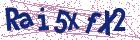# Estimation of P[Y < X] for generalized exponential distribution in presence of outlier

Document Type : Research Article

Authors

Abstract

This paper deals with the estimation of P(Y < X), where Y has generalized exponential distribution with parameters a and A and X has mixture gen-eralized exponential distribution (or marginal distribution of X\, X2,…. Xn,
in presence of one outlier with parameters ß1 and ß2 ) such that X and Y are independent, when the scale parameter (A) is known the maximum like-lihood estimator of R = P(Y < X) is derived. Analysis of a simulated data
set has also been presented for illustrative purposes.

Keywords20.1001.1.24236977.2009.2.1.7.9